# Class 12 Physics: CBSE Sample Question Paper- Term I (2021-22)- 2 Notes | Study Physics Class 12 - NEET

## NEET: Class 12 Physics: CBSE Sample Question Paper- Term I (2021-22)- 2 Notes | Study Physics Class 12 - NEET

The document Class 12 Physics: CBSE Sample Question Paper- Term I (2021-22)- 2 Notes | Study Physics Class 12 - NEET is a part of the NEET Course Physics Class 12.
All you need of NEET at this link: NEET

Class-XII
Time: 90 Minutes
Max. Marks: 35

General Instructions :

1. The Question Paper contains three sections.
2. Section A has 25 questions. Attempt any 20 questions.
3. Section B has 24 questions. Attempt any 20 questions.
4. Section C has 6 questions. Attempt any 5 questions.
5. All questions carry equal marks.
6. There is no negative marking.
Section - A

This section consists of 25 multiple choice questions with overall choice to attempt any 20 questions. In case more than desirable number of questions are attempted, ONLY first 20 will be considered for evaluation.

Q.1: Charging occurs due to
(a) transfer of electrons
(b) transfer of protons
(c) transfer of neutrons
(d) None of these

Since electron is about 1800 times lighter than proton, so on applying an electric field electron will be accelerated fast. Additionally, electrons are the mobile charge and can be free to move if sufficient energy to give, whereas protons are bound to the nuclei. So, cause of charging is actual transfer of electrons.

Q.2: Torque acting on a dipole having dipole moment vector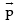in a uniform electric field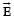is
(a)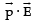(b)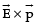(c)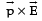(d) zero

Torque τ on a dipole in a uniform electric field = force × perpendicular distance.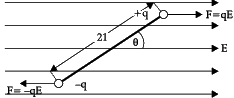τ= qE( 2l sinθ ) = 2qlE sinθ = pE sinθ
Where,    p = 2ql  Nm.
In vector form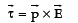Q.3: Along an electric line of force, electric potential
(a) increases
(b) decreases
(c) remains same
(d) infinite

Since
dV = -E.ds

⇒ (Vf - Vi) = -E.ds

⇒ (Vi - Vf) = -E.ds

This indicates that on moving along the line of force V decreases as V>V.

Q.4: The capacitance between two plates of capacitor increases with
(a) increase the plate area and decrease the distance between the plates.
(b) decrease the plate area and increase the distance between the plates.
(c) decrease the plate area and distance between the plates.
(d) increase the plate area and distance between the plates.

Capacitance of parallel plate capacitor is given by: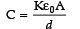where, C = capacitance of parallel plate capacitor
K = dielectric constant of dielectric material
A = area of each plate
d = distance between the plates
Therefore, C increases as A increases and d decreases

Q.5: Work done by moving a charged particle from one point to another in an electrostatic field __________ upon the path chosen.
(a) depends
(b) does not depend
(c) may or may not depend
(d) None of these

Work done by moving a charge q from a point P1 having potential V1 to another point P2 having potential V2 is:
W = q (V2 – V1)
In terms of force and displacement, work done is: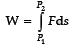=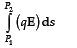=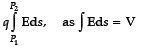=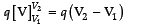The equation indicates that whatever path is chosen from point P1 to point P2, the integral will have only one value.

Q.6: When cell of e.m.f. E is connected with an external resistance R, the potential difference across the cell becomes V. The internal resistance of the cell is:
(a)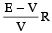(b)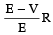(c)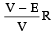(d)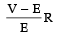Current in the circuit = I = V/R If r = internal resistance of the cell,
then V = E  – Ir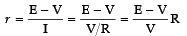Q.7: When a current drawn from a cell increase, the potential difference across the terminal of cell is
(a) lowered

(b) more
(c) remain same
(d) infinite

Let the internal resistance of cell = r and current drawn from it  = i. Then, the terminal potential difference V = E – Ir Since, i is increasing, the terminal potential difference will decrease.

Q.8: When the wire carrying current is charged
(a) never
(b) Always
(c) At the time of switch on
(d) At the time of switch off

Electric current is the flow of electron and in current carrying wire, as many electrons enter at one end, the same number of electrons leave at other end. Therefore, current carrying wire is never charged.

Q.9: In the presence of an electric field, paths of electrons in metals are ________ between two successive collisions.
(a) straight in one direction
(b) straight in two directions
(c) curved
(d) none of these

It is curved because there is motion of charge in an electric field.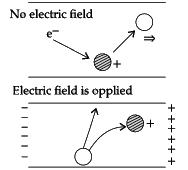Q.10: Example of a non-ohmic resistance is:
(a) copper wire
(b) tungsten wire
(c) diode
(d) carbon resistor

According to ohm’s law, current flowing through a conductor is directly proportional to the applied potential difference across the conductor. For diode, expression of current is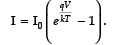Thus, current is not directly proportion to applied potential difference. Hence, diode is non-ohmic.

Q.11: Kirchhoff’s second law of electrical circuit is based on
(a) conservation of energy
(b) conservation of charge
(c) conservation of momentum
(d) none of these

Kirchhoff’s second law states that in any closed loop of a mash, the sum of potential difference across the components is zero. In other word, total energy gained per unit charge is equal to the energy lost per unit charge. Therefore, this law is based on the conservation of energy.

Q.12: Two parallel, long wires carry currents i1 and i2 with i1 > i2. When the current are in the same direction, the magnetic field at a point midway between the wire is 10 mT. If the direction of i2 is reversed, the field becomes 30 mT. The ratio i1/i2 is.
(a) 4
(b) 3
(c) 2
(d) 1

B = B– B2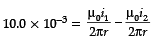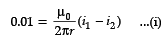In 2nd case
B = B1 + B2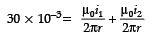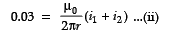e.q. (i) divide by eq. (ii)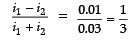3(i1 – i2) = i1  + i2
3i1  – 3i2 = i1  + i2
3i1  – i1  = i2 + 3i2
2i1  = 4i2
i1 / i2 = 4 / 2
i1 / i2 = 2

Q.13: The magnetism of a magnet is due to
(a) spin of electrons
(b) the earth
(c) cosmic rays
(d) pressure of big magnet inside the earth

The magnetism of a magnet is produced due to spin of electrons. Each electron is revolving around the nucleus of an atom. The revolving electron is equivalent to a tiny loop of current and exhibit orbital magnetic dipole moment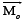. Additionally, electron spins around its axis. Another dipole moment is there, which is know as spin magnet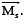the net magnetic dipole moment is the vector sum of these, i.e.,+Q.14: The magnetic field around a long straight current carrying wire is
(a) spherical symmetry
(b) cubical symmetry
(c) cylindrical symmetry
(d) unsymmetrical

Magnetic field is given by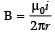i.e. B ∝ 1/r, which indicates that magnetic field around a long straight current carrying wire is cylindrical symmetry.

Q.15: An electron moves in a circular path with a uniform speed v. It produces magnetic field at the center of the circle. The radius of the circle is proportional to
(a)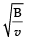(b) B/v
(c)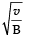(d) v/B

Here, centripetal force is provided by magnet force, i.e.,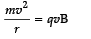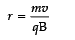r ∝ v/B

Q.16: A moving coil has N number of turns in a coil of effective area A, it carries a current I. Produced magnetic field is radial. the torque acting on the coil is given by
(a) NIBA
(b) N2IBA
(c) NI2BA
(d) NIB2A

Torque is given by τ = NIBA sinθ

Since the magnetic field is radial; θ = 90°
⇒τ = NIBA

Q.17: In a moving coil galvanometer, the deflection of the coil θ is related to the current i
(a) i ∝ tanθ
(b) i ∝ θ

(c) i ∝ √θ
(d) i ∝ θ2

Moving coil galvanometer is worked on the principle that when a current carrying coil is placed in magnetic field, it experiences a torque. Since torque is proportional to the current, therefore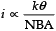. This states that deflection of the coil is directly proportion to current.

Q.18: The instantaneous flux  associated with a closed circuit of 10 W resistance is indicated  by the following reaction f = 6t2 – 5t + 1, then value in amperes of the induced current at t = 0.25 sec will be:
(a)1.2
(b) 0.8
(c) 6
(d) 0.2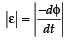|ε| = |-(12t - 5)|
|ε| = 12t - 5
at 0.25 Sec
|ε| = 12(0.25) - 5
|ε| = 2

∴ ε / R = 2 / 10 = 0.2 A

Q.19: In electromagnetic induction, the induced charge is independent of
(a) time
(b) change of flux
(c) resistance of coil
(d) None of these.

Since, e = dφ/dt,
but, e = iR and i = dq/dt
⇒ dq/dt .R = dφ/dt
⇒ dq = dφ /R
This clearly states that electromagnetic induction, the induced charge depends only on change of flux and resistance of coil, and independent from time.

Q.20: A dynamo converts
(a) mechanical energy into thermal energy.
(b) Thermal energy into mechanical energy.
(c) mechanical energy into electrical energy.
(d) electrical energy into mechanical energy.

A dynamo is an electrical generator that produces direct current with the use of a commutator. It is capable of delivering power in many places like industries, factories and foundation upon which many other electric-power conversion devices were based. Thus, dynamo converts mechanical energy into electrical energy.

Q.21: Figure represents an area A = 2 m2 situated in a uniform magnetic field B = 4 weber/m2 and making an angle of 60° with magnetic field lines. The magnetic flux through the area would be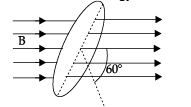(a) 4 weber
(b) 8 weber
(c) 4√3 weber
(d) 2√2 weber

φ= BAcosθ
= 4 x 2 x cos60°
= 4 x 2 x 1 / 2
= 4 weber

Q.22: A.C power is transmitted from a power house at a high voltage as
(a) the rate of transmission is faster at high voltages.
(b) the rate of transmission is faster at low voltages.
(c) power cannot be transmitted at low voltages.
(d) it is more economical due to power loss.

Here, power loss i.e., V2/R and i2R occurs in form of heat. For long distance, length of wire is large and have very high resistance R ∝ l . Therefore, there is a loss in power as resistance is inversely proportion to power loss. So, it is more economical due to power loss.

Q.23: Power in an A.C. circuit is rated per second at which
(a) charge flows
(b) energy spends
(c) current changes alternatively
(d) work is done

Power = Work/time = W/t

Q.24: Which of the following represents the correct graph of inductive reactance XL and frequency f.
(a)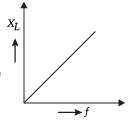(b)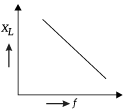(c)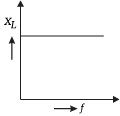(d)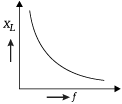Since,
XL = ωL = 2πfL
⇒ XL = f

Q.25: When an AC voltage of 220 V is applied to the capacitor C
(a) the current is in phase with the applied voltage.
(b) the charge on the plates is in phase with the applied voltage.
(c) the maximum voltage between plates is 220 V.
(d) power delivered to the capacitor is infinite.

When an AC voltage of 220 V is applied to the capacitor C, the charge on the plates is in phase with the applied voltage.

Section - B
This section consists of 24 multiple choice questions with overall choice to attempt any 20 questions. In case more than desirable number of questions are attempted, ONLY first 20 will be considered for evaluation.

Q.26: If a unit positive charge is taken from one point to another over an equipotential surface, then :
(a) Work is done on the charge
(b) Work is done by the charge
(c) Work done is constant
(d) No work is done

It is observed that on equipotential surfaces, electric field results as normal to the charged surface not allowing the work done.

Q.27: The electric field inside a spherical shell of uniform surface charge density is :
(a) Zero
(b) Constant, less than zero
(c) Directly proportional to the distance from the centre
(d) None of the above

In spherical shell, the electric field is zero as in a shell every charge lies on outer surface, so from Gauss law, electric field inside a shell will be zero.

Q.28: The distance between the two charges +q and −q of a dipole is r. On the axial line at a distance d from the centre of dipole, the intensity is proportional to :
(a) q/d2
(b) qr/d2
(c) q/d3
(d) qr/d3

It is observed that field along the axis of dipole will be:
E = (1/4πε0) × 2p/d3 = (1/4πε0) × 2(q×r)/d3
With this, E ∝ qr/d3

Q.29: 2μF capacitance has potential difference across its two terminals 200 volts. It is disconnected from battery and then another uncharged capacitance is connected in parallel to it, then P.D. becomes 20 volts. Then the capacity of another capacitance will be :
(a) 2 μF
(b) 4 μF
(c) 18 μF
(d) 10 μF

On applying common potential:
V = (C1V1 + C2V2)/C1 + C2
Now 20 = (2 × 200 + C2 × 0)/(2 + C2)
Capacity of second capacitance will be C2 = 18 μF

Q.30: A charge Q is placed at the corner of a cube. The electric flux through all the six faces of the cube is :
(a) Q/3 ε0
(b) Q/6 ε0
(c) Q/8 ε0
(d) Q/ε0

According to the Gauss’ theorem, the electric flux through a close surface in Q/ε0 .

Q.31: The capacity of a parallel plate capacitor with no dielectric substance but with a separation of 0.4 cm is 2 μF. The separation is reduced to half and it is filled with a dielectric substance of value 2.8. The final capacity of the capacitor is :
(a) 11.2 μF
(b) 15.6 μF
(c) 19.2 μF
(d) 22.4 μF

The capacitance of parallel plate capacitor:
C = ε0KA/d
Now    C1/C2 = (K1/K2) × (d2/d1)
Further 2/C2 = (1/2.8)×[(0.4/2)/(0.4)]
On solving, we see that C2 = 11.2μF

Q.32: A short bar magnet of magnetic moment 1 J/T is placed with its axis at 60° to a uniform magnetic field of 0.2 T. Calculate the magnitude of the torque experience.
(a) 3 × 10-1 Nm
(b) √3 × 10-2 Nm
(c) 3 × 10-2 Nm
(d) √3 × 10-1 Nm

M = 1 J/T, q = 60°, B = 0.2 T
τ = MB sin q
= 1 × 0.2 × sin 60
=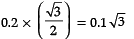= √3 × 10-1 Nm

Q.33: In the adjoining circuit, the e.m.f. of the cell is 2 volt and the internal resistance is negligible. The resistance of the voltmeter is 80 ohm. The reading of the voltmeter will be :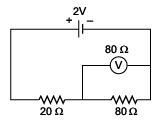(a) 0.80 volt
(b) 1.60 volt
(c) 1.33 volt
(d) 2.00 volt

From the above circuit, total resistance = (80/2) + 20 = 60 Ω
The main current i = 2/60 = (1/30) A
Now, the voltage across voltmeter and 80 Ω resistance.
V =R′ × i= 40 x 1/30 V = 1.33V

Q.34: Through two parallel wires A and B, 10A and 2A of currents are passed respectively in opposite directions. If the wire A is infinitely long and the length of the wire B is 2 m, the force on the conductor B, which is situated at 10 cm distance from A will be :
(a) 8 × 10−5 N
(b) 4 × 10−7 N
(c) 4 × 10−5 N
(d) 4π × 10−7 N

Force on the conductor B:  F = (μ0/4π) × 2 × (i1i2/a) × l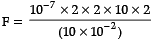= 8 × 10−5 N

Q.35: If a long hollow copper pipe carries a current, then magnetic field is produced
(a) inside the pipe only
(b) outside the pipe only
(c) both inside and outside the pipe
(d) no where

According to Ampere’s circuital law, B(2πr)=μo × 0 or B = 0 as I = 0 (inside) So, inside a hollow metallic pipe carrying current, the magnetic field is zero. But for external points, the whole current behaves as if it were concentrated at the axis only. Thus, the magnetic field is produced outside the pipe only.

Q.36: If the current is halved in a coil, then the energy stored is how much times the previous value?
(a) 1/2
(b) 1/4
(c) 2
(d) 4

Energy stored is given as, U = (½) × Li2
Also, U2/U1 = (i2/i1) 2 = (1/2)2 = 1/4
U2 = (¼) × U1

Q.37: In a circuit L, C and R are connected in series with an alternating voltage source of frequency f. The current leads the voltage by 45°. The value of C is :
(a) 1/2πf(2πfL+R)
(b) 1/πf(2πfL+R)
(c) 1/2πf(2πfL−R)
(d) 1/πf(2πfL−R)

Now, it is known that,
tan φ = (XC − XL)/R
=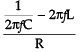So tan 45o = [(1/2πfC)−2πfL]/R
Hence        C = 1/[2πf(2πfL+R)]

Q.38: The natural frequency of a L-C circuit is equal to :
(a)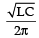(b)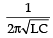(c)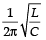(d)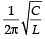In an LC circuit, resonance takes place when the circuit is driven from external source at angular frequency ω0 where inductive and capacitive reactance are equal in magnitude.
XL = XC

ω0L = 1/ω0C
or, ω0 = 1/√LC
or, 2πf0 = 1/√LC
or, f0 = 1/ 2π√LC

Q.39: An ac source is connected to a resistive circuits. Which of the following is true?

(a) Current leads the voltage and both are in same phase
(b) Current lags behind the voltage and both are in same phase
(c) Current and voltage are in same phase
(d) Any of the above may be true depending upon the value of resistance

In a purely resistive circuit, all circuit power is dissipated by the resistor(s). Voltage and current are in phase with each other.

Q.40: What will be the direction of induced current when the current in the vertical wire is reducing at a steady rate?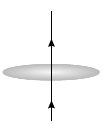(a) anti-clockwise
(b) Clockwise
(c) No current will be induced
(d) None of the above

No current will be induced since the field lines are lying in the plane of the closed loop.

Q.41: Given the resistances of 1 Ω, 2 Ω, 3 Ω. How will these be combined to get an equivalent resistance of 11/5Ω?
(a) 1Ω and 2Ω in parallel and with this combination 3Ω in series.
(b) 3Ω and 2Ω in parallel and with this combination 1Ω in series.
(c) 1Ω and 3Ω in parallel and with this combination 2Ω in series.
(d) all three resistors in parallel

When 3Ω and 2Ω are in parallel, the equivalent resistance is

R23 =6/5Ω
When 1Ω and R23 are joined in series, the equivalent resistance is

Req = 11/5Ω

Q.42: Three capacitors each of capacitance 9 pF are connected in series and then connected to a 120 V supply.
The total capacitance of the combination is ________ and the potential difference across each capacitor is ________ .
(a) 27pF, 120V
(b) 3 pF, 40V
(c) 27pF, 40V
(d) 3pF, 120V

Capacitance of each of the three capacitors is 9 pF.
Equivalent capacitance = Ceq
1/Ceq = 1/9 +1/9+1/9
∴  Ceq = 3pF
Supply voltage, V=120 V Potential difference (V’) across each capacitor is equal to one-third of the supply voltage i.e. 40V.

Q.43: Capacitance of a single conductor means
(a) When connected to a supply one side of the conductor will be positively charged, other side will be negatively charged.
(b) It is a parallel plate capacitor with two plates coinciding with each other
(c) It is a parallel plate capacitor. One plate is the conductor and the other plate is at infinite distance.
(d) None of the above.

Q.44: An arbitrary surface encloses a dipole. Electric flux through this surface is
(A) 0
(B) ∞
(C) Depends on the surface enclosing the dipole.
(D) Depends on the dipole moment

By Gauss’ theorem of electrostatics, the flux crossing a closed surface is equal to the net charge enclosed by the surface divided by ε0.

In case of a dipole, net charge inside the Gaussian surface is + q – q = 0 Since the net charge of a dipole is zero, the total flux crossing the surface is also zero.

Given below are two statements labelled as Assertion (A) and Reason (R)
Directions: In the following questions, a statement of Assertion (A) and is followed by a statement of Reason (R). Mark the correct choice as:
(a) Both Assertion (A) and Reason (R) are true, and Reason(R) is the correct explanation of (A).
(b)
Both Assertion (A) and Reason (R) are true, but Reason (R) is not the correct explanation of Assertion (A).
(c)
Assertion (A) is true, but Reason (R) is false.
(d)
Assertion (A) is false, but Reason (R) is true.
Q.45:

Assertion (A): In a cavity in a conductor, the electric field is zero.
Reason (R): Charges in a conductor reside only at its surface.

If the magnet moves quicker, the rate of change of flux is also higher, hence the EMF induced is more in case (i).

Q.46:

Assertion (A): Capacity of a conductor is independent on the amount of charge on it.
Reason (R): Capacitance depends on the dielectric constant of surrounding medium, shape and size of the conductor.

C = Aε0/d
In the expression, there is no involvement of charge. So, capacitance is independent of charge. Hence the assertion is true.
It depends on permittivity of the surrounding medium and the area of the plate. So, reason is also true.
Reason explains the assertion.

Q.47:

Assertion (A): Galvanometer to ammeter conversion takes place by connecting a low value resistance in parallel with it.
Reason (R): The low value resistance increases the effective resistance and protects the galvanometer.

Galvanometer to ammeter conversion  takes place by connecting a low value resistance known as “shunt” in parallel with it. The assertion is true.
When two resistors are connected in parallel then the effective resistance  becomes lower than the lowest value of the two resistors. Hence the reason is false.

Q.48:

Assertion (A): Self inductance may be called the inertia of electricity.
Reason (R): Due to self inductance, opposing induced e.m.f. is generated in a coil as a result of change in current or magnetic flux linked with the coil.

Inertia is defined as the tendency of an object to resists its change of state of motion. Induced e.m.f. in a coil is changed by the change in current or magnetic flux. The property by which a coil opposes these parameters to incur any change in induced e.m.f. is known as selfinductance. Hence, self inductance may be called the inertia of electricity. So, the assertion and reason both are true but reason cannot explain why so happens.

Q.49:

Assertion (A): A transformer does not work on DC.

Reason (R): DC neither change direction nor magnitude.

Transformer has two coils. If current fluctuates in one coil, e.m.f. is induced in the other coil. For DC supply current does not change, so there is no induced e.m.f. Hence both assertion and reason are true and reason explains the assertion.

Section - C
This section consists of 6 multiple choice questions with an overall choice to attempt any 5. In case more than desirable number of questions are attempted, ONLY first 5 will be considered for evaluation.

Q.50: A small object with charge q and weight mg is attached to one end of a string of length ‘L’ attached to a stationary support. The system is placed in a uniform horizontal electric field ‘E’, as shown in the accompanying figure. In the presence of the field, the string makes a constant angle θ with the vertical. The sign and magnitude of q: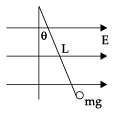(a) positive with magnitude mg/E
(b) positive with magnitude (mg/E)tan θ
(c) negative with magnitude mg/E tan θ
(Dd) positive with magnitude E tan θ/mg

Fe = mg tan θ
qE = mg tan θ
q =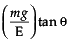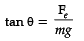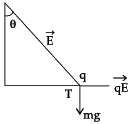Q.51: A free electron and a free proton are placed between two oppositely charged parallel plates. Both are closer to the positive plate than the negative plate.
Which of the following statements is true?
I. The force on the proton is greater than the force on the electron.
II. The potential energy of the proton is greater than that of the electron.
III. The potential energy of the proton and the electron is the same.

(a) I only
(b) II only
(c) III and I only
(d) II and I only

∵ Fp = Fe
F = qE
E = same
'q' = same
Now, Pε = q V(r)
(P. ε)p > (P . ε)e

CASE STUDY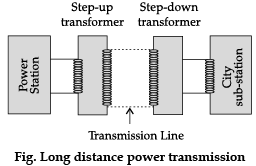The large-scale transmission and distribution of electrical energy over long distances is done with the use of transformers. The voltage output of the generator is stepped-up. It is then transmitted over long distances to an area sub-station near the consumers. There the voltage is stepped down. It is further stepped down at distributing sub-stations and utility poles before a power supply of 240 V reaches our homes.
Q.52: Which of the following statement is true?
(a) Energy is created when a transformer steps up the voltage
(b) A transformer is designed to convert an AC voltage to DC voltage
(c) Step–up transformer increases the power for transmission
(d) Step–down transformer decreases the AC voltage

Correct answer is (D) i.e. step down transformer decreases the ac voltage.

Q.53: If the secondary coil has a greater number of turns than the primary,
(a) the voltage is stepped-up (Vs >Vp) and arrangement is called a step-up transformer
(b) the voltage is stepped-down (Vs <Vp) and arrangement is called a step-down transformer
(c) the current is stepped-up (Is > Ip) and arrangement is called a step-up transformer
(d) the current is stepped-down (Is < Ip) and arrangement is called a step-down transformer

i.e. Ns/Np = Es/Ep
i.e. if number of turns in secondary coil are more than number of turns in primary, then voltage is increased or stepped up in secondary, so called step- up transformer.

Q.54: We need to step-up the voltage for power transmission, so that
(a) the current is reduced and consequently, the I2R loss is cut down
(b) the voltage is increased, the power losses are also increased
(c) the power is increased before transmission is done
(d) the voltage is decreased so V2/R losses are reduced

i.e. Current is reduced if voltage is stepped-up, so corresponding I2R losses are cut down.

Q.55: A power transmission line feeds input power at 2300 V to a step down transformer with its primary windings having 4000 turns. The number of turns in the secondary in order to get output power at 230 V are
(a) 4
(b) 40
(c) 400
(d) 4000

Given
E= 2300 V
E0 = 230 V

Np = 4000
Ns = ?
Ei/Eo = Np/Ns
2300/230 = 4000/x
x = 400 = Ns = number of turns is secondary coil.

The document Class 12 Physics: CBSE Sample Question Paper- Term I (2021-22)- 2 Notes | Study Physics Class 12 - NEET is a part of the NEET Course Physics Class 12.
All you need of NEET at this link: NEETUse Code STAYHOME200 and get INR 200 additional OFF

## Physics Class 12

134 videos|388 docs|213 tests

### How to Prepare for NEET

Read our guide to prepare for NEET which is created by Toppers & the best Teachers

Track your progress, build streaks, highlight & save important lessons and more!

,

,

,

,

,

,

,

,

,

,

,

,

,

,

,

,

,

,

,

,

,

;